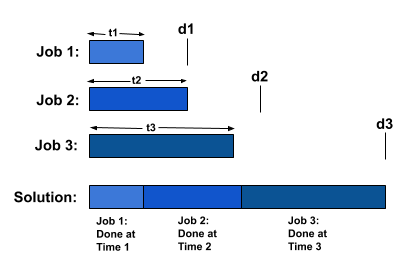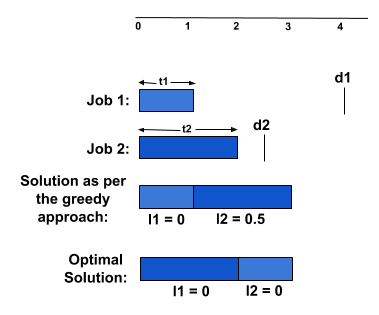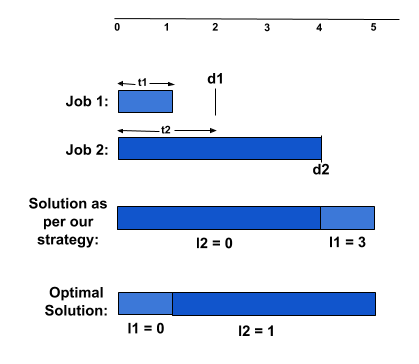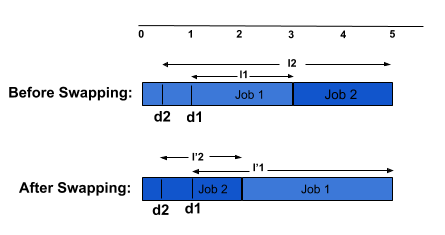×

Search anything:

# Scheduling tasks to Minimize Lateness

#### Algorithms Greedy AlgorithmsGet this book -> Problems on Array: For Interviews and Competitive Programming

Reading time: 25 minutes | Coding time: 5 minutes

### The Problem

As they say, "Greed... is good. Greed is right. Greed works". We will look into one such problem where greedy proves its worth. According to the problem, We have a single resource and a set of n requests to use the resource for an interval of time. Each request i has a deadline di and time required to finish the request ti. Each request i must be assigned an interval of time ti which must not overlap with other accepted requests. Also, one must note that since we are scheduling the requests on one resource, we could find the starting(si) and finishing time(fi) of each by the relation fi = si + ti. We are willing to schedule maximum requests before their respective deadlines or at least in a way to decrease the time lag in finish time and deadline of the chosen request (i.e., lateness).

We claim a request i to be late if fi > di. If a request finishes before its deadline, we consider its lateness(li) to be zero and fi - di otherwise.

We are interested to find a schedule such that the maximum lateness (max(li*) where i ∈ {1...n}) is minimized.

Consider below example, we are given 3 requests(i=3) along with the time required to complete each one(ti) and their respective deadlines(di). We could schedule the requests in many ways but we choose the shown solution since it fulfills our motive to complete all the requests within the assigned deadlines.In this case, since every request managed to finish before its deadline, the lateness of every request is 0 and hence the maximum lateness is also 0, which is minimum.

In above example, we could intuitively see the solution but how to proceed when n is sufficiently large? Here comes the need to define an Algorithm that will give the expected solution in almost every situation.

### Greedy Strategies

A Greedy Algorithm works in stages and at every stage, it makes the best local choice depending on the past ones and assures to give a globally optimal solution.

Needless to say, there would be many greedy approaches that will give optimal solution in certain situations but our task is to find one that will work in every situation. Hence, we will now see if we could get an efficient greedy algorithm for our problem.

# Shortest Processing Time First

With a little thought, one could claim a strategy which sorts the Processing time in ascending order will give us optimal solution since this way, we would be able to finish the smaller requests quickly. What do you think?
Yes, it won't work for us! It doesn't consider the deadlines of requests and this is where it looses the sprint.You can clearly see that according to this strategy, we will choose t1 instead of t2 due to its lesser processing time but t2 will miss its deadline this way contributing towards a positive lateness which could have been 0 if we scheduled t2 before t1 (refer to the image above).
So, this strategy fails.

# Minimum Slack Time First

Coming to the next strategy, we would like to consider the deadlines while scheduling. A solution could be considering the difference between the deadline and its processing time to choose a request(i.e., slack time) and adding requests with increasing slack time. This do works in the above scenario though. But it too fails. Check the example shown below:Here as the slack time of t2 is smaller than t1 (0<1), we scheduled it first but as we could note, it leads to lateness of 3 in t1 and 0 in t2.Hence, calling the maximum latency as 3 in our solution. But if we consider the optimal solution, we can clearly see that this leads to lateness of 1 in t2 and 0 in t1 which means the maximum lateness here is 1. Therefore, this greedy algorithm fails.

Let's see another strategy which is based on choosing requests with increasing deadline. Surprisingly, it gives an optimal solution to our problem and we couldn't find a contradicting case here like above.
Quick Challenge - Check if this works fine in previous two examples.

# Proving Optimality

We can't claim our greedy algorithm to be efficient due to lack of contradicting cases. So, we will rather prove that this gives an optimal solution to our problem.
We will consider that there exist an optimal solution O and the A is the solution returned by the greedy algorithm.
Since proving optimality of an algorithm is no easy task, We will gradually transform O into a schedule that is identical to A making sure that it holds optimality in every step. This procedure is generally called as an "Exchange Argument".
To Prove: "The schedule A produced by our greedy strategy has optimal maximum lateness".

Proof: We will first prove that "All schedules with no inversions and no idle time have the same maximum lateness".

where we say, there is an Inversion if there exist two job i, j such that di > dj and i is scheduled before j and Idle time is the time lag when the resource is not working yet there are requests which need to be scheduled.

If there are two different schedules which have neither inversions nor idle time, then they might differ by the order in which the requests are scheduled in them. Since we have scheduled the requests in increasing order of deadline, we can be assured that all the jobs with deadline less than di are scheduled before i and those with greater deadline after i.
Therefore, the only possibility of having different order in schedules is due to the requests with same deadline. Consider the requests with deadline d, the last request with deadline d will have the maximum lateness and our maximum lateness depends on this request irrespective of the order of jobs.It supports our claim that "All schedules with no inversions and no idle time have the same maximum lateness".

Next up, we will prove that "There is an optimal schedule that has no inversions and no idle time"
Note that for every Optimal schedule O, we can always shift our requests in order to eliminate the idle time as there is no constraint on the starting time but on the finish time.
Also, let's assume that O has an inversion, i.e., there are two requests i, j with di < dj and j is scheduled before i. We could swap ith and jth request and hence can decrease the number of inversion. But we are left to prove that the new schedule after eliminating the inversion maintains optimality.
Let lk be the lateness of requests before swapping (where k ∈ {i, j}) and l'k be the lateness of requests after swapping.Note that d2<d1 but 1 is scheduled before 2. Hence there is an inversion. After swapping, we should observe that:
l'1 = f'1 - d1
= f2 - d1 (since f'1 = f2)
<= f2 - d2 (d2 < d1)
<= l2
And we just saw that maximum lateness doesn't increase after swapping a pair with adjacent inversion.

Now, we have sufficient information to prove "The schedule A produced by the greedy algorithm has optimal maxmum lateness L"
As we discussed above, we know that there exist an Optimal schedule O that has no inversion. Recall that by choosing our greedy strategy (Earliest Deadline First) we will never get any inversions in our schedule. Moreover, we have proved that all the schedules with no inversions have the same maximum lateness.
Hence, the schedule obtained by the greedy algorithm is optimal.

The Pseudocode for the algorithm could be written as:

``````1. Sort the requests by their deadline
2. min_lateness = 0
3. start_time = 0
4. for i = 0 -> n
5.     min_lateness = max(min_lateness, (t[i] + start_time) - d[i])
6.     start_time += t[i]
7. return min_lateness;
``````

### Implementation

``````#include <bits/stdc++.h>
using namespace std;

class Request
{
public:
bool operator < (const Request & x) const
{
}
};
int main()
{
int n, i, finish_time, start_time, min_lateness, temp;
cout << "Enter the number of requests: ";
cin >> n; // no. of requests
Request r[n];
cout << "Enter the deadline and processing time of each request: ";
for (i = 0; i < n; i++) // deadline and processing time of each job
sort(r, r + n); // sort jobs in increasing order of deadline
start_time = 0;
min_lateness = 0;
for (i = 0; i < n; i++)
{
min_lateness = max((r[i].p_time + start_time) - r[i].deadline, min_lateness);
start_time += r[i].p_time;
}
cout << "Maximum lateness of schedule: " <<  min_lateness;
return 0;
}
``````

### Complexity

• Time complexity: `Θ(N log N)` due to sorting (We could reduce the Time Complexity to `Θ(N)` if the requests are already sorted

• Space complexity: `Θ(1)`

With this article at OpenGenus, you must have complete idea of scheduling tasks to minimize delay. Enjoy.

#### Shweta Bhardwaj

I panic and hence I exist | Intern at OpenGenus | Student at Indraprastha College for Women, University of Delhi